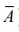Home | | Business Maths 11th std | Basic concepts of Probability

# Basic concepts of Probability

Descriptive statistics and probabilityExample: Tossing a coin, throwing a die, selecting a card from a pack of playing cards, etc.

Basic concepts of Probability

Recall

### (i) Random Experiment

If an experiment or trial can be repeated under the same conditions, any number of times and it is possible to count the total number of outcomes, but individual result ie., individual outcome is not predictable, then the experiment is known as random experiment.

Example: Tossing a coin, throwing a die, selecting a card from a pack of playing cards, etc.

### (ii) Outcome:

The result of a random experiment will be called an outcome.

### (iii) Trial and Event:

Any particular performance of a random experiment is called a trial and outcome or combinations of outcomes are termed as events.

### (iv)  Exhaustive Events:

The total number of possible outcomes of a random experiment is known as the exhaustive events.

### (v) Favourable Events:

The number of cases favourable to an event in a trial is the number of outcomes which entail the happening of the event.

### (vi) Mutually Exclusive events:

Events are said to be mutually exclusive if the happening of any one of them precludes the happening of all the others, ie., if no two or more of them can happen simultaneously in the same trial. Symbolically the event A and B are mutually exclusive if A Ōł® B = Ōłģ .

### (vii) Equally Likely Events:

Events (two or more) of an experiment are said to be equally likely, if any one of them cannot be expected to occur in preference to the others.

### (viii) Classical definition of Probability

If a random experiment or trial results in ŌĆśnŌĆÖ exhaustive, mutually exclusive and equally likely outcomes (or cases), out of which m are favourable to the occurrence of an event E, then the probability ŌĆśpŌĆÖ of occurrence (or happening) of E, usually denoted by P(E), is given byProperties

(i) 0ŌēżP(E)Ōēż1

(ii) Sum of all the probability equal to 1.

(iii) If P(E)=0 then E is an impossible event.

For example : A coin is tossed. Find the probability of getting a head

Solution: The total possible outcomes of an experiment {H,T}

Therefore n = 2

The favourable outcome for getting a head {H}. Therefore m =1. Thus the required probability is### (x) Modern Definition of Probability

The modern approach to probability is purely axiomatic and it is based on the set theory concepts. In order to study, the theory of probability with an axiomatic approach it is necessary to define certain basic concepts. They are

(i) Sample Space: Each possible outcome of an experiment that can be repeated under similar or identical conditions is called a sample point and the collection of sample points is called the sample space, denoted by S.

(ii) Event: Any subset of a sample space is called an event.

(iii) Mutually Exclusive events:  Two events A and B are said to be mutually exclusive events if A Ōł® B = ŽĢ i.e., if A and B are disjoint sets.

Example: Consider        S       = { 1,2,3,4,5}

Let     A = the set of odd numbers     = {1,3,5}

and    B = the set of even numbers    = {2,4}

Then           AŌł®B = ŽĢ

Therefore the events A and B are mutually exclusive

### (xi) Observation:

Statement meaning in terms of Set theory approach### (xii) Definition of Probability (Axiomatic approach)

Let E be an experiment. Let S be a sample space associated with E. With every event in S we associate a real number denoted by P(A) called the probability of the event A satisfying the following axioms.

Axiom 1 :  P(A)Ōēź0

Axiom 2 :  P(S)=1

Axiom 3     :  If A1 , A2 ,......, An  be a sequence of n mutually exclusive events in then

P ( A1 UA2UŌĆ”UAn ) = P ( A1 )+ P ( A2 )+ ŌĆ”+ P ( An )

### (xiii) Basic Theorems on probability

Theorem 1:

Then P( Ōłģ ) = 0 i.e., probability of an impossible event is zero.

Theorem 2:

Let S be the sample space and A be an event in S then P() ŌēĪ 1ŌĆō P(A)

If A and B are any two events then P ( A Ōł¬ B ) = P( A ) + P( B ) ŌłÆ P( A Ōł® B)

### (xiv) Observation:

(i) If the two events A and B are mutually exclusive then AŌł®B = Ōłģ

Ōł┤P(AŌł®B) = 0

ŌćÆP(AUB) = P(A) + P(B)

(ii) The addition theorem may be extended to any three events A,B,C and we have

P ( AŌł¬ B Ōł¬ C) = P ( A) + P ( B)+ P ( C) ŌłÆ P ( AŌł® B ) ŌłÆ P( A Ōł® C) ŌłÆ P ( B Ōł® C) + P ( AŌł® BŌł®C)

It is believed that the students might be familiar with the above concepts and our present syllabus continues from the following.

Tags : Probability , 11th Business Mathematics and Statistics(EMS) : Chapter 8 : Descriptive statistics and probability
Study Material, Lecturing Notes, Assignment, Reference, Wiki description explanation, brief detail
11th Business Mathematics and Statistics(EMS) : Chapter 8 : Descriptive statistics and probability : Basic concepts of Probability | Probability